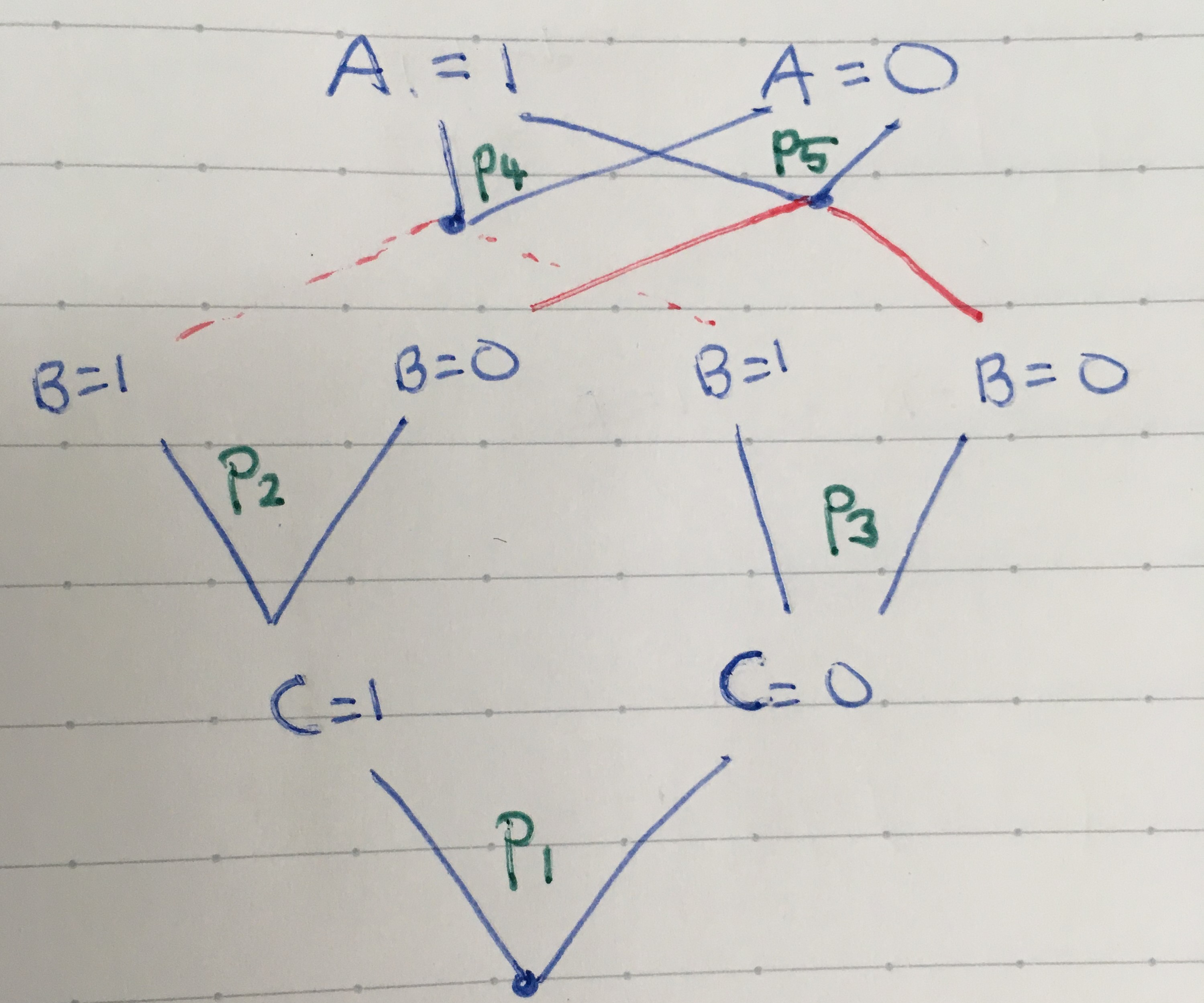Show Question

# How many parameters are needed to describe this distribution?

Consider a distribution consisting of three binary variables which admit the factorisation: $$p(a, b, c) = p(a \mid b)p(b \mid c)p(c)$$

How many parameters are needed to specify distributions of this form?

Note that this is different to the general case where all variables might be dependent: $$p(a, b, c) = p(a \mid b, c)p(b \mid c)p(c)$$

In the case where we have no information about the variable dependency, we need to specify 8-1=7 parameters to define the distribution. There are $$2^3 = 8$$ possible outcomes, but as the probabilities add to 1, we only need 7 parameters to fully define the distribution.

In the given case, b's value is sufficient to know the distribution of a. So some simplification is possible. A way to think about the situation is to consider 5 coins: $$c, {b_1, b_2}, {a_1, a_2}$$. We will flip 3 coins starting with coin $$c$$. The outcome of flipping $$c$$ determines with coin $$b$$ from $${b_1, b_2}$$ will be flipped. The outcome of flipping coin $$b$$ determines which coin $$a$$ from $${a_1, a_2}$$. As the coins can be represented as bernoilli random variables, they can be described with a single parameter representing the probability of landing heads. As there are 5 coins, we need 5 parameters to fully describe the distribution of the whole system.

Drawing a graph is another way to model this problem.## Source

Bayesian Reasoning and Machine Learning
David Barber
Q 1.6